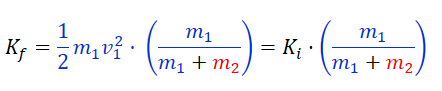top of page
Search

# Inelastic collisions: The physics of snowball fights!Key concepts:

Inelastic collision

Conservation of Momentum

Conservation of Energy

Question:

"A 1 kg snowball is moving with 10m/s. It collides inelastically with another ball (your head!) that has a mass of 10kg. Determine how much energy is lost in the collision." To get full marks, we need the following:

1. A sketch/cartoon showing the two balls before, during and after the collision.

2. The total momentum before the collision.

3. The total momentum after the collision.

4. The speed of the object formed by the collision.

5. The total kinetic energy before the collision.

6. The total kinetic energy after the collision.

Let's get started with 1.

1. A sketch/cartoon showing the two balls before, during and after the collision.

To make the drawing correctly we have to remember what an "inelastic collision" means. In short:

A collision is "inelastic" if the objects fuse together when they crash into each other. The "kinetic energy" is NOT CONSERVED!

An everyday example could be a soft snowball colliding with your face.

The other case is that of "elastic" collisions:

A collision is "elastic" if the objects simply bounce off of each other when they touch. The "kinetic energy" is CONSERVED!

With this in mind, it is easy to make the drawing correctly.

Before:During:After:As you can see, it is a good idea to use colors and to write down symbols for all the information involved. Here, we have used:In physics problems, it is often useful to use the subscript "i" for the "initial" speed/mass/temperature etc., and "f" for the "final" value.

Now we are ready to tackle 2.

• 2. The total momentum before the collision.

The momentum before the collision can be calculated from:Think of "momentum" as meaning "the total amount of stuff that is moving".

Let's look at the "before" drawing. Clearly only the mass m1 is moving at the speed v1=10m/s . The other mass, m2 is stationary, so v2=0m/s.Great! Now we can tackle 3.

• 3. The total momentum after the collision.

Here, we can use something called "Conservation of Momentum". This principle basically says that "The amount of stuff moving around" in the beginning is the same as "The amount of stuff moving around" in the end. Mathematically, we say thatIn the end we have one combined object of mass M with moving at a speed vf.

What is equal to? Well we have not destroyed the masses m1 or m2 . We have just fused them together. Therefore the total mass isUsing this information, we can figure out 4.

• 4.The speed of the object formed by the collision.

We write down the law of conservation of momentum and solve for vf. The final speed of M is:Take a look at this mathematical expression. It says that the final speed, vf, is some number, m1/(m1+m2), times the initial speed, v1. Do you think the final speed is bigger or smaller than the initial speed? Let us check by plugging in the numbers:It looks like the final speed is smaller than the initial speed. That kind of makes sense, right? We are throwing a light object onto a heavy one, so they stick together. Throwing a snowball at a train will only cause it to move a little bit.

Now let us think about 5.

• 5. The total kinetic energy before the collision.

Remember that kinetic energy of a single object is defined as:So the total kinetic energy before the collision must beOf course the second mass has no energy, because it is not moving. By plugging in the numbers we have, we find:Now let us take a look at 6.

• 6. The total kinetic energy after the collision

Before we calculate this, do you think the kinetic energy after the collision is bigger or smaller than the initial amount? Let us plug in the expressions for M and vf:Take the square of everything inside the parenthesis:Cancel out the (m1+m2) in the top and bottom:If we are smart, we can rewrite this a little bit more:Now we can easily see that the final kinetic energy is some number times the initial kinetic energy. From 4. we know thatTherefore the final kinetic energy is:So in this inelastic collision, we lost around 45J out of the 50J we had in the beginning!